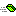## IComparable

Defines a generalized type-specific comparison method that a value type or class implements to order or sort its instances.

### Public:

#### Methods:

NameDescriptionCompareTo Compares the current instance with another object of the same type and returns an integer that indicates whether the current instance precedes, follows, or occurs in the same position in the sort order as the other object.

#### Remarks

This interface is implemented by types whose values can be ordered or sorted. It requires that implementing types define a single method, CompareTo(Value), that indicates whether the position of the current instance in the sort order is before, after, or the same as a second object of the same type. The instances IComparable implementation is called automatically by methods such as CorArray.Sort and ArrayList.Sort.

The implementation of the CompareTo(Value) method must return a 32-bit integer that has one of three values, as shown in the following table.

ValueMeaning
Less than zeroThe current instance precedes the value specified by the CompareTo method in the sort order.
ZeroThis current instance occurs in the same position in the sort order as the value specified by the CompareTo method.
Greater than zeroThis current instance follows the object specified by the CompareTo method in the sort order.

All number types (such as Long and Double) are supported by the default Comparer, as are String and Date. Custom types should also provide their own implementation of IComparable to enable object instances to be ordered or sorted.

#### Example

The following example illustrates the implementation of IComparable and the requisite CompareTo method.

There are two modules created for this example. A BAS module containing the Main method and a Class module implementing the IComparable interface.

The following is a Visual Basic Class module named Temperature.

```Option Explicit
Implements IComparable

Private mTemperatureF As Double

Public Function CompareTo(ByRef Value As Variant) As Long
Dim Other As Temperature

On Error GoTo Catch
Set Other = Value

If Other Is Nothing Then
CompareTo = 1
Else
CompareTo = Comparer.Default.Compare(mTemperatureF, Other.Fahrenheit)
End If
Exit Function

Catch:
Throw NewArgumentException("Value is not a Temperature")
End Function

Public Property Get Fahrenheit() As Double
Fahrenheit = mTemperatureF
End Property

Public Property Let Fahrenheit(ByVal Value As Double)
mTemperatureF = Value
End Property

Public Property Get Celsius() As Double
Celsius = (mTemperatureF - 32) * (5# / 9#)
End Property

Public Property Let Celsius(ByVal Value As Double)
mTemperatureF = (Value * 9# / 5#) + 32
End Property

'''''''''''''''''''''''''''''''''''''''''''''''''''''''''''''
'   IComparable
'''''''''''''''''''''''''''''''''''''''''''''''''''''''''''''
Private Function IComparable_CompareTo(Value As Variant) As Long
IComparable_CompareTo = CompareTo(Value)
End Function
```

The following is a Visual Basic BAS module containing Main start-up method.

```Public Sub Main()
Dim Temperatures As New ArrayList
Dim r As New Random
Dim i As Long
Dim Degrees As Double
Dim Temp As Temperature

For i = 1 To 10
Degrees = r.NextRange(0, 100)
Set Temp = New Temperature
Temp.Fahrenheit = Degrees
Next

Temperatures.Sort

For Each Temp In Temperatures
Debug.Print Temp.Fahrenheit
Next
End Sub

' This code produces the following output (individual values may vary because they are randomly generated):
'
'    13
'    18
'    31
'    36
'    65
'    65
'    66
'    78
'    92
'    98
```